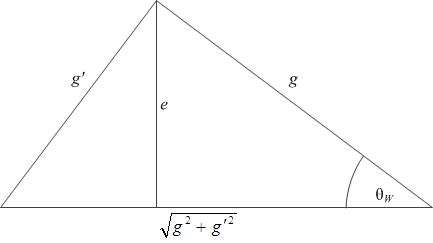Electroweak theory has several coupling constants: there is g, there is g‘, there is e, and then there is the Weinberg angle θW and its sine and cosine, and I am always worried about making mistakes.

Well, here’s a neat way to remember: the three constants and the Weinberg angle have a nice geometrical relationship (as it should be evident from the fact that the Weinberg angle is just a measure of the abstract rotation that is used to break the symmetry of a massless theory).This diagram also makes it clear that so long as you keep the triangle a right triangle, all it takes is two numbers (e.g., e and θW) and the triangle is fully determined. This is true even when the coupling constants are running.